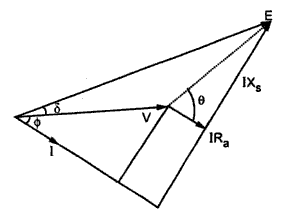Theory of cylindrical rotor machine

Let us consider the phasor diagram for alternator as shown in the Fig. 1.Fig. 1

Let          E = E.M.F. induced in each phase
V = Terminal voltage
Φ = Phase angle between voltage and current
δ = Power angle
R= Resistance of armature
X= Synchronous reatance of alternator.
...                   tanθ = Xs/R
...                   θ = tan-1 (Xs/Ra)
α = Φ + δ
Power output per phase = V . I cos (V ^ I)
= VI cos
Power input,   Pi = EI cos (E ^ I)
= E I cos (Φ + δ) = E I cosα = I (E cos α)
= I (V cos Φ + I Ra)
= VI cosΦ + I2 R
The voltage equation of alternator is given by,

Pis the power converted internally into electrical power from mechanical power which is given by,
P= Eo . I

Note : With increase in δ power increases and with decrease in δ power decreases. Power in case of synchronous machines depends on the angle δ. This angle δ is called power angle.
In case of large synchronous machines,  Xs >>> R
...                θ = tan-1(Xs/R)= 90o (if resistance is neglected)
θ = 90o
Substituting this value in above expression for power